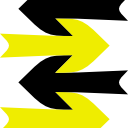Phil’s Math Assignments

home
math
general rules The world is understood by physics, which depends on the power of math and computers. Math and computers relate to logic.
 upcoming matrix & vector practice upcoming convert a complex number to the matrix representing the same possibility lecture on length and perpendicularity of vectors

Past assignments:

Course Overview

• 2004-09 to 2005-06
• Described as Pre-Calculus
but we know better!
• There will be more matrices and combinatorics.
• There will be plenty of logarithms, exponentials, sines, tangents, and functions.
• There will be complex numbers, polynomials, roots, and factoring.
• But we've seen all of those before! So we will be doing all of these things while beginning to learn calculus.
• Plus, there will be more programming, logic, and proofs. The presentation will be unusual, because your teacher is still trying to reinforce his weak intuition for the Curry-Howard Isomorphism and for Category Theory. So the programming language will have explicit logic, also known as static types. It could be Haskell or even Dad's own Ambidexter, if that gets off the ground. There's this nifty book, The Haskell Road to Logic, Maths and Programming, which has an introduction to many wonderful things, but unfortunately it seems to be weak on the Curry-Howard Isomorphism as well.
• For Calculus, the prime textbook candidate is the classic, by Thomas.
• For Pre-Calculus, the prime textbook is Algebra and Trigonometry, by Paul A. Foerster.

Support open standards!